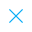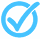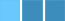Call Support +91-85588-96644

Keep me logged in
You can't leave Captcha Code empty
By submitting this form, you agree to the Terms & Privacy Policy.
ORTests given

OR# HLT - 3 (Mathametics Class - X)

Topics Covered:Trigonometric Ratios of Complementary AnglesTrigonometric Ratios of Standard AnglesCalculating Mean from its DefinitionCalculating Median from a Frequency Distribution TableConstructing and Interpreting OgivesDerivation of Other Complex IdentitiesBasic Trigonometric IdentitiesCalculating Mean by Step Deviation MethodCalculating Mode from a Frequency Distribution TableCalculating Other Trigonometric Ratios When One is Given

This pdf is currently unavailable.